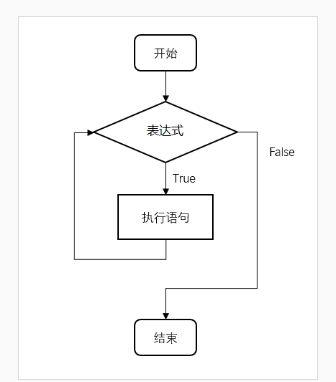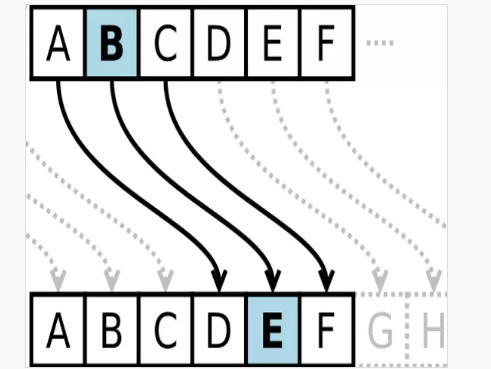• 循环结构
python循环结构
1.1 使用while
Python 中没有 do…while 循环
while … else 在条件语句为 false 时执行 else 的语句块：
list = [1,2,3,4,5]
i = 0

while i < len(list):
print(list[i])
i += 1

1
2
3
4
5
# while可以使用else语句
list = [1,2,3,4,5]
i = 0
while i < len(list):
print(list[i])
i += 1
else :
print('**** 程序执行结束 *****')

1
2
3
4
5
**** 程序执行结束 *****
1.2 使用for

for循环可以遍历任何序列的项目，如一个列表或者一个字符串。

一般结构如下
for <variable> in <sequence>:
<statements>
else:
<statements>

# 遍历数字， 可以使用range方法
for i in range(6):
print(i)
# 第二个参数不可达，第三个参数为步长不指定时，默认为1
for i in range(2,6,2):
print(i)

0
1
2
3
4
5
2
4


展开全文• 遍历结构&amp;amp;gt;: 语句块 种类 - 计数循环 for &amp;amp;lt;循环变量&amp;amp;gt; in range(N[,M[,K]]): 语句块 字符串遍历循环 s表示字符串，c是字符串中的字符 for
目录

遍历循环
无限循环
循环的保留字
循环的高级用法

遍历循环
表达方式
for <循环变量> in <遍历结构>:
语句块

种类

计数循环

for <循环变量> in range(N[,M[,K]]):
语句块


字符串遍历循环

s表示字符串，c是字符串中的字符
for c in s:
语句块


列表遍历循环

for item in ls:
语句块


文件遍历循环

fi表示文件的标识符，line表示文件中的每一行
for line in fi:
语句块

无限循环<条件循环>
表达式，直到条件不满足的时候，才不执行语句块
while <条件>：
语句块

循环控制的保留字
break和continue是python在循环控制中的保留字

break 跳出当前循环，并且执行循环后面的语句
continue 结束当前循环，继续执行后续次数循环

如果循环有多层怎么办呢

break 一个break仅仅只能跳出一层循环
continue 仍然是结束当前层当前循环，继续执行后续次数循环

循环的高级用法
while循环和else语句的结合

当循环没有被break退出时，可以执行else中的语句块
else语句块可以看作是“正常”完成循环的奖励

for <循环变量> in <遍历结构>:
语句块
else:
语句块

while <条件>：
语句块
else:
语句块



展开全文遍历
• ## 1.6 Python循环结构

万次阅读 2019-05-18 12:48:26
1.6 Python循环结构1.6 Python循环结构1.6.1 while型循环1.6.2 for ... in 循环1.6.3 range()函数:1.6.4 break和continue语句及循环中的else子句： 1.6 Python循环结构 循环结构就是为了将相似或者相同的代码...
1.6 Python循环结构1.6 Python循环结构1.6.1 while型循环1.6.2 for ... in 循环1.6.3 range()函数:1.6.4 break和continue语句及循环中的else子句：
1.6 Python循环结构

循环结构就是为了将相似或者相同的代码操作变得更见简洁，使得代码可以重复利用

循环结构分为2类：while循环 和 for…in循环

1.6.1 while型循环
格式1：
while 条件表达式：
循环的内容
[变量的变化]

格式2：

while 条件表达式：
循环的内容
[变量的变化]
else:
python语句..


注意:while循环中的else是在while条件表达式为假的情况下执行的代码内容,一般用于判断起始条件是否为假等相关操作。

实例使用了 while 来计算 1 到 100 的总和：
#!/usr/bin/env python3

n = 100
sum = 0
counter = 1
while counter <= n:
sum = sum + counter
counter += 1

print("1 到 %d 之和为: %d" % (n,sum))


执行结果如下：

1 到 100 之和为: 5050

死循环：

死循环就是循环不会终止的循环类型，通过将用于判断的条件表达式设置为永远为True来实现。

    while True:
python代码...
python代码...
...

#!/usr/bin/python3

var = 1
while var == 1 :  # 表达式永远为 true
num = int(input("输入一个数字  :"))
print ("你输入的数字是: ", num)

print ("Good bye!")


你可以使用 CTRL+C 来退出当前的无限循环
执行以上脚本，输出结果如下：

输入一个数字  :5
你输入的数字是:  5
输入一个数字  :

1.6.2 for … in 循环

for…in 循环用于遍历容器类的数据（字符串，列表，元组，字典，集合）

格式：

for  变量  in  容器：
python代码,可以在此使用变量

格式2：

for 变量1,变量2 in 容器：
python代码,可以在此使用变量1和变量2


要求遍历的容器必须是一下几种格式：

[(),(),()] 列表中有元组
[[],[],[]] 列表中有列表
((),(),()) 元组中有元组
{(),(),()} 集合中有元组
字典的特殊使用

格式3：
for  变量  in  容器：
python代码,可以在此使用变量
else:
循环结束是执行的代码！

>>>languages = ["C", "C++", "Perl", "Python"]
>>> for x in languages:
...     print (x)
...
C
C++
Perl
Python
>>>

1.6.3 range()函数:

如果你需要遍历数字序列，可以使用内置range()函数。它会生成数列，例如:

>>>for i in range(5):
...     print(i)
...
0
1
2
3
4

>>>for i in range(5,9) :
print(i)

5
6
7
8
>>>

>>>for i in range(0, 10, 3) :
print(i)

0
3
6
9
>>>

>>>for i in range(-10, -100, -30) :
print(i)

-10
-40
-70
>>>


您可以结合range()和len()函数以遍历一个序列的索引,如下所示:

>>>a = ['Google', 'Baidu', 'Runoob', 'Taobao', 'QQ']
>>> for i in range(len(a)):
...     print(i, a[i])
...
1 Baidu
2 Runoob
3 Taobao
4 QQ
>>>

输出乘法口诀：
for i in range(1,10):
for j in range(1,i+1):
print(str(i)+'*'+str(j)+"="+str(i*j),end="")
print()

1.3.7 作业：逆向输出乘法口诀：
for i in range(9,0,-1):
for j in range(i,0,-1):
print(str(i)+'*'+str(j)+"="+str(i*j),end="")
print()

1.6.4 break和continue语句及循环中的else子句：
break语句：

break作用:在循环中break的作用是终止当前循环结构的后续操作，一旦程序运行了break，循环也就终止了！
break 语句可以跳出 for 和 while 的循环体。如果你从 for 或 while 循环中终止，任何对应的循环 else 块将不执行。 实例如下：

#!/usr/bin/python3

for letter in 'Runoob':     # 第一个实例
if letter == 'b':
break
print ('当前字母为 :', letter)

var = 10                    # 第二个实例
while var > 0:
print ('当期变量值为 :', var)
var = var -1
if var == 5:
break

print ("Good bye!")


执行以上脚本输出结果为：

当前字母为 : R
当前字母为 : u
当前字母为 : n
当前字母为 : o
当前字母为 : o
当期变量值为 : 10
当期变量值为 : 9
当期变量值为 : 8
当期变量值为 : 7
当期变量值为 : 6
Good bye!

continue语句：

continue语句被用来告诉Python跳过当前循环块中的剩余语句，然后继续进行下一轮循环。

#!/usr/bin/python3

for letter in 'Runoob':     # 第一个实例
if letter == 'o':        # 字母为 o 时跳过输出
continue
print ('当前字母 :', letter)

var = 10                    # 第二个实例
while var > 0:
var = var -1
if var == 5:             # 变量为 5 时跳过输出
continue
print ('当前变量值 :', var)
print ("Good bye!")


执行以上脚本输出结果为：

当前字母 : R
当前字母 : u
当前字母 : n
当前字母 : b
当前变量值 : 9
当前变量值 : 8
当前变量值 : 7
当前变量值 : 6
当前变量值 : 4
当前变量值 : 3
当前变量值 : 2
当前变量值 : 1
当前变量值 : 0
Good bye!

循环语句可以有 else 子句，它在穷尽列表(以for循环)或条件变为 false (以while循环)导致循环终止时被执行,但循环被break终止时不执行。
如下实例用于查询质数的循环例子:

#!/usr/bin/python3

for n in range(2, 10):
for x in range(2, n):
if n % x == 0:
print(n, '等于', x, '*', n//x)
break
else:
# 循环中没有找到元素
print(n, ' 是质数')


执行以上脚本输出结果为：

2  是质数
3  是质数
4 等于 2 * 2
5  是质数
6 等于 2 * 3
7  是质数
8 等于 2 * 4
9 等于 3 * 3

pass 语句:

Python pass是空语句，是为了保持程序结构的完整性。

pass 不做任何事情，一般用做占位语句，如下实例

>>>while True:
...     pass  # 等待键盘中断 (Ctrl+C)
最小的类:

>>>class MyEmptyClass:
...     pass



展开全文笔记
• 文章目录Educoder—第3章 Python 循环结构第1关：Python循环结构之while循环生成温度转换表第2关：Python循环结构之while循环实现凯撒密码加密第3关：Python循环结构之while循环打印九九乘法表第4关：Python循环结构...
文章目录Educoder—第3章 Python 循环结构第1关：Python循环结构之while循环生成温度转换表第2关：Python循环结构之while循环实现凯撒密码加密第3关：Python循环结构之while循环打印九九乘法表第4关：Python循环结构之for循环实现奇偶数统计第5关：Python循环结构之for循环实现阶乘计算第6关：Python循环结构之break之判断素数第7关：Python循环结构之break第8关：Python循环结构之continue第9关：Python循环结构之continue之求正奇数个数第10关：Python循环结构之for…else…之寻找水仙花数第11关：Python循环结构之for…else…
Educoder—第3章 Python 循环结构
本章目标是通过学习具备编写 Python 循环结构程序的能力，涉及的 Python 编程基本概念包括：字符串数据类型（ string ）的基本概念，循环语句 while、for、for-else，break、continue 语句，range 的使用和列表的基本概念等。
第1关：Python循环结构之while循环生成温度转换表任务：构造-20到50摄氏度的摄氏-华氏温度对应表。
本关的摄氏温度值都为整数。温度表中的温度跨度为1度。数字与—之间有一个空格。
输出格式：
摄氏度—华氏度
-20 —> -4.000000
-19 —> -2.200000
···
···
50 —> 122.000000
min_temp = -20     # 最小温度值
max_temp = 50     # 最大温度值
c = -20
print("摄氏度--->华氏度")     # 打印出表的第一格
while c<51:
f = c*1.8+32
print("{} ---> {:.6f}".format(c,f))
c = c + 1

执行结果：
D:\网络安全\Python\py_code>python educ.py
摄氏度--->华氏度
-20 ---> -4.000000
-19 ---> -2.200000
-18 ---> -0.400000
-17 ---> 1.400000
-16 ---> 3.200000
-15 ---> 5.000000
-14 ---> 6.800000
-13 ---> 8.600000
-12 ---> 10.400000
-11 ---> 12.200000
-10 ---> 14.000000
-9 ---> 15.800000
-8 ---> 17.600000
-7 ---> 19.400000
-6 ---> 21.200000
-5 ---> 23.000000
-4 ---> 24.800000
-3 ---> 26.600000
-2 ---> 28.400000
-1 ---> 30.200000
0 ---> 32.000000
1 ---> 33.800000
2 ---> 35.600000
3 ---> 37.400000
4 ---> 39.200000
5 ---> 41.000000
6 ---> 42.800000
7 ---> 44.600000
8 ---> 46.400000
9 ---> 48.200000
10 ---> 50.000000
11 ---> 51.800000
12 ---> 53.600000
13 ---> 55.400000
14 ---> 57.200000
15 ---> 59.000000
16 ---> 60.800000
17 ---> 62.600000
18 ---> 64.400000
19 ---> 66.200000
20 ---> 68.000000
21 ---> 69.800000
22 ---> 71.600000
23 ---> 73.400000
24 ---> 75.200000
25 ---> 77.000000
26 ---> 78.800000
27 ---> 80.600000
28 ---> 82.400000
29 ---> 84.200000
30 ---> 86.000000
31 ---> 87.800000
32 ---> 89.600000
33 ---> 91.400000
34 ---> 93.200000
35 ---> 95.000000
36 ---> 96.800000
37 ---> 98.600000
38 ---> 100.400000
39 ---> 102.200000
40 ---> 104.000000
41 ---> 105.800000
42 ---> 107.600000
43 ---> 109.400000
44 ---> 111.200000
45 ---> 113.000000
46 ---> 114.800000
47 ---> 116.600000
48 ---> 118.400000
49 ---> 120.200000
50 ---> 122.000000

第2关：Python循环结构之while循环实现凯撒密码加密任务：编写代码实现凯撒密码加密，密码只能为大写字母，原密码和偏移量都通过input函数获取。
需要考虑边界效应，如果密码超过了Z，则重新用A、B、C…表示。
需要用到的内置函数
chr 函数
chr(x) 使用一个整数x作为参数，返回参数x对应的 ASCII 码字符
ord 函数
ord 函数是 chr 函数的配对函数，ord(ch)使用一个字符ch作为参数，返回ch对应的 ASCII 的十进制数值。
key = str(input("please input the key:"))
p = int(input("please input the offset:"))
i = 0
chkey = ""

while i<len(key):
#偏移后的ASCII
num = ord(key[i])+p
#若偏移后的大于90，90就是Z，就要从A开始继续轮回，即考虑边界效应
if num > 90:
num  = num-90+64
string =  chr(num)
chkey += string
i = i+1
print(chkey)

执行结果：
D:\网络安全\Python\py_code>python eee.py
MQKPQ

D:\网络安全\Python\py_code>python eee.py
DEFG

第3关：Python循环结构之while循环打印九九乘法表
任务：使用while循环打印出九九乘法表。注意每行算式间有一个空格，每一行的末尾有一个空格，乘号使用的是 x。
说明：print(“Hello Python”, end="\n")，print()函数默认带换行参数，将其等于空就不会换行了
i = 1
while i<10:
j = 1
while j<(i+1):
res = i*j
print("{}x{}={} ".format(i,j,res),end="")
j+=1
i+=1
print()

执行结果：
D:\网络安全\Python\py_code>python educ.py
1x1=1
2x1=2 2x2=4
3x1=3 3x2=6 3x3=9
4x1=4 4x2=8 4x3=12 4x4=16
5x1=5 5x2=10 5x3=15 5x4=20 5x5=25
6x1=6 6x2=12 6x3=18 6x4=24 6x5=30 6x6=36
7x1=7 7x2=14 7x3=21 7x4=28 7x5=35 7x6=42 7x7=49
8x1=8 8x2=16 8x3=24 8x4=32 8x5=40 8x6=48 8x7=56 8x8=64
9x1=9 9x2=18 9x3=27 9x4=36 9x5=45 9x6=54 9x7=63 9x8=72 9x9=81

第4关：Python循环结构之for循环实现奇偶数统计
任务：分别统计给定的列表中奇数和偶数的个数。
对range函数的说明

range()函数的使用
​		range(n)	表示一个区间范围的递增的数据	[0, n)
​		range(m, n)	表示一个区间范围，[m, n)的区间
​		range(m, n, s)	第三个值表示步长step
帮助文档
>>> help(range)
Help on class range in module builtins:

class range(object)
|  range(stop) -> range object
|  range(start, stop[, step]) -> range object
|
|  Return an object that produces a sequence of integers from start (inclusive)
|  to stop (exclusive) by step.  range(i, j) produces i, i+1, i+2, ..., j-1.
|  start defaults to 0, and stop is omitted!  range(4) produces 0, 1, 2, 3.
|  These are exactly the valid indices for a list of 4 elements.
|  When step is given, it specifies the increment (or decrement).
翻译一下

>>>帮助（范围）

模块内置中有关类范围的帮助：

类范围（对象）

|范围（停止）->范围对象

|范围（开始，停止[，步骤]）->范围对象

|

|返回一个对象，该对象从一开始就生成一个整数序列（包括）

|一步一步地停止（排除）。范围（i，j）产生i，i+1，i+2，…，j-1。

|开始默认为0，停止被忽略！范围（4）产生0、1、2、3。

|这些正是4个元素列表的有效索引。

|当给定step时，它指定增量（或减量）。


"""
任务：分别统计给定的列表中奇数和偶数的个数。
"""
list_value = [1, 2, 18, 7, 33, 22, 1045, 98, 78, 36, 10, 111, 105, 4320, 1014, 50, 63, 15, 18, 910, 2010, 3201, 2501, 25, 120, 320]

ji = 0
ou = 0
for i in range(len(list_value)):
if list_value[i]%2 == 0:
ou +=1
else:
ji += 1
print("奇数共{}个，偶数共{}个".format(ji,ou))

执行结果;
D:\网络安全\Python\py_code>python educ.py
奇数共11个，偶数共15个

第5关：Python循环结构之for循环实现阶乘计算
任务：使用 for 循环计算某个正整数的阶乘，这个数通过 input 函数获取。
如果输入的数不为正整数，则输出“输入数据不合法”。
注意：0 的阶乘为 1。
num = int(input())
times = 1
flag = 1
if num < 0:
flag = 0
for i in range(1,num+1):
flag = 1
if num == 0:
times = 1
break
else:
times *= i
if flag == 1:
print("{}的阶乘为{}".format(num,times))
else:
print("输入数据不合法")

执行结果:
D:\网络安全\Python\py_code>python educ.py
5
5的阶乘为120

D:\网络安全\Python\py_code>python educ.py
4
4的阶乘为24

D:\网络安全\Python\py_code>python educ.py
0
0的阶乘为1

D:\网络安全\Python\py_code>python educ.py
-7
输入数据不合法


第6关：Python循环结构之break之判断素数
“”"
任务：判断给定的整数是否是素数。该整数是通过 input 获取。如果输入的数小于1，则输出“输入数据不合法”。
“”"
什么是素数？

素数是指在大于 1 的自然数中，除了 1 和它本身以外不再有其他因数的自然数

num = int(input())
i = 2
flag = 1
if num < 1:
print("输入数据不合法")
while i<num:
if num%i == 0:
flag = 0
break
i += 1
if flag == 0:
print("不是素数")
elif flag == 1 and num > 0:
print("是素数")

执行结果;
D:\网络安全\Python\py_code>python educ.py
15
不是素数

D:\网络安全\Python\py_code>python educ.py
11
是素数

D:\网络安全\Python\py_code>python educ.py
0
输入数据不合法

第7关：Python循环结构之break
“”"
任务：模仿银行输入的密码出错 3 次，而锁定账户(三次重新输入密码的机会)，
请编写代码实现该功能。密码通过 input 函数获取。正确密码为"123"。
如果密码错误，输出一次“密码错误”，如果密码正确，输出“密码正确”。
“”"
flag = 1
for i in range(3):
print("密码正确")
break
else:
print("密码错误")

执行结果：
D:\网络安全\Python\py_code>python educ.py
123
密码正确

D:\网络安全\Python\py_code>python educ.py
345
密码错误
567
密码错误
678
密码错误

D:\网络安全\Python\py_code>python educ.py
345
密码错误
123
密码正确

第8关：Python循环结构之continue
“”"
任务：计算给定列表中所有正数的和。
“”"
list_num = [-5, 0, 5, 8, 101, -16, -15, 56, 20, -32, 20, 0, -19, 50, 100, 205, 302, 1901, 302]
sum = 0
for i in range(0,len(list_num)):
if list_num[i] <= 0:
continue
else:
sum += list_num[i]
print("正数和为{}".format(sum))

执行结果：
D:\网络安全\Python\py_code>python educ.py
正数和为3070

第9关：Python循环结构之continue之求正奇数个数
“”"
任务：给定一个正整数 n，统计小于等于 n 的正奇数的个数。n 通过 input 获取。如果输入的数据小于或者等于 0，则输出“输入数据不合法”。
“”"
num = int(input())
res = 0
flag = 0
#注意：当num小于0时，是不符合range()函数的可迭代对象的，即不进入for循环中
for i in range(1,num+1):
if i%2 == 0:
continue
res += 1
flag = 1
if flag == 0:
print("输入数据不合法")
else:
print("正奇数个数为{}".format(res))

执行结果：
D:\网络安全\Python\py_code>python educ.py
15
正奇数个数为8

D:\网络安全\Python\py_code>python educ.py
-5
输入数据不合法

D:\网络安全\Python\py_code>python educ.py
100
正奇数个数为50

第10关：Python循环结构之for…else…之寻找水仙花数
相关知识
针对本关任务，我们可以直接使用循环结构和分支结构语句相配合完成，不过，在这里，将介绍 for-else 语句来完成本关任务。
for-else
for-else 语句是 for 循环的一种，它和 break 语句一起使用时才能显示出 else 的作用。下面给出了两个使用 for-else 的例子及其执行结果。
for i in range(10):
if i == 5:
print( 'found it! i = %s' % i)
else:

执行结果：
found it! i = 5

这段代码中，在i=5时使用了break结束了循环
for i in range(10):
if i == 5:
print( 'found it! i = %s' % i)
break
else:

执行结果：
found it! i = 5

从上述两段代码的运行结果可知，第一段代码并没有使用 break 语句，循环是完整的进行完了的，所以 else 语句中的代码运行了，而在第二段代码中，在 i=5 时使用了 break 结束了循环，所以 else 语句中的代码并没有执行。可以发现，如果循环在运行过程中被中断，则 else 语句不会执行；只有当循环完整的运行结束，else才会去执行。
“”"
任务：给定一个整数 n，判断 n 以内的正数是否含有水仙花数，n 通过 input 获取;
如果有，输出“有水仙花数”，如果没有，则输出“没有水仙花数”。
水仙花数：一个 3 位的整数，它的每个位上的数字的 3 次幂之和等于它本身。
“”"
from math import *
n = int(input())
#由于水仙花数是三位的整数，所以范围限制在了100-999；所以for循环从100开始
for i in range(100,n):
gewei = i%100%10
shiwei = i%100//10
beiwei = i//100
res = pow(gewei,3) + pow(shiwei,3) + pow(beiwei,3)
if res == i:
print("有水仙花数")
#break使循环中断后将不会执行else语句
break
else:
#当for循环完整的执行完成后，即没有发现水仙花数，就会执行else语句
print("没有水仙花数")

执行结果：
D:\网络安全\Python\py_code>python educ.py
123
没有水仙花数

D:\网络安全\Python\py_code>python educ.py
800
有水仙花数

第11关：Python循环结构之for…else…
“”"
任务：给定一个正整数 n，这个正整数通过 input 获取，统计 2 到 n 的素数个数是否超过了 10 个。
如果超过了 10 个，则输出“素数数量超过10个”，如果少于 10 个，则输出“素数数量少于10个”，如果输入的数是 0 或 1，则输出“输入数据不合法”。
“”"
n = int(input())

#判断输入是否合法
if n == 0 or n == 1:
print("输入数据不合法")
else:
#num用于迭代2到输入的数字
for num in range(2,n+1):
if num == 2:
else:
#内层循环用于统计，2-n中每一个数字是不是素数
for i in range(2,num):
#素数是不能被整除的,,如果可以被整除，肯定不是素数
if (num%i) == 0:
break
else:
print("素数数量超过10个")
break
else:
print("素数数量少于10个")

执行结果：
D:\网络安全\Python\py_code>python educ.py
30
素数数量少于10个

D:\网络安全\Python\py_code>python educ.py
57
素数数量超过10个

D:\网络安全\Python\py_code>python educ.py
0
输入数据不合法

D:\网络安全\Python\py_code>python educ.py
60
素数数量超过10个



展开全文• ## Python循环结构语句

千次阅读 2020-05-27 15:48:20
1、for循环：计次循环 2、while循环：条件循环 3、嵌套循环 4、跳转语句 ...小编创建了一个Python学习交流QQ群：531509025 寻找有志同道合的小伙伴，互帮互助,群里还有不错的视频学习教程和PDF电子书！ ''' &
• ## Python系列7-Python循环结构for语句

千次阅读 热门讨论 2021-03-24 13:41:30
需要对列表中的每个元素都执行相同的操作时，可使用Python中的for 循环。 1.1 从一个案例来看for循环 我这边有一个当前市面上流行的数据库的列表，我需要将这个列表所有的值都打印出来 代码: dbs = ['Oracle','MySQL...列表 for
• ## python循环结构 练习

千次阅读 2019-08-15 17:00:07
循环结构 –for - in 循环 如果明确的知道循环执行的次数或者是要对一个容器进行迭代（后面会讲到），那么我们推荐使用for-in循环。 #用于设定for循环的迭代次数 #range也是一个前闭后开 #可迭代对象 for x in range......

# python循环结构python 订阅